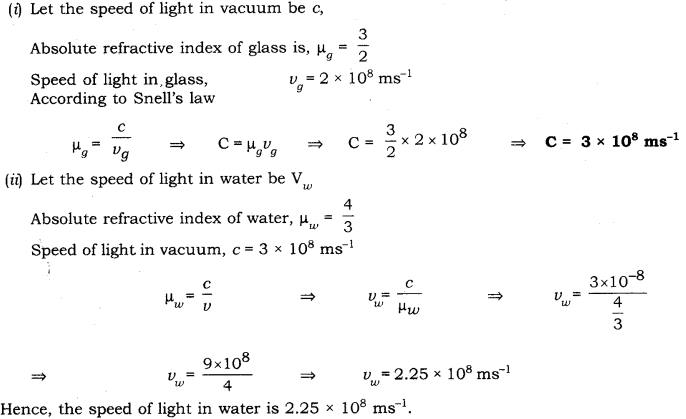# The absolute refractive indices of glass and water are 4/3 and 3/2 respectively

The absolute refractive indices of glass and water are 4/3 and 3/2 respectively. If the speed of light in glass is 2×108 m/s, calculate the speed of light in
(i) vacuum
(ii) water.